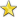# Heroic Tokens

edited September 2016
What is the probability that you get a 2, 3or 4cover from a heroic token? The in-game description gives a chance of 0.2% chance to get one of 35 4covers, a chance of 0.6% to get one of 40 3covers and a chance of 5.4% to get one of 13 2covers. Simply adding these numbers you get a chance of 70.2%+24%+7%=101.4% to get any cover which is clearly wrong and indicates that the numbers are rounded.
If we assume that the numbers are rounded to one decimal after the comma, we get that the chance to get a 2cover is in the interval [69.55, 70.85), the chance to get a 3cover is in the interval [22, 26) and the chance to get a 4cover is in the interval [5.25, 8.75). If we assume even numbers, the probabilities could be (70%, 22%, 8%), (70%, 23%, 7%) or (70%, 24%, 6%). Does anyone know the real numbers?